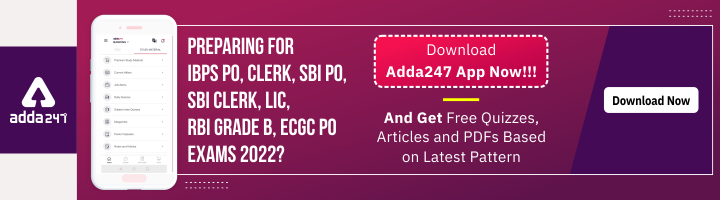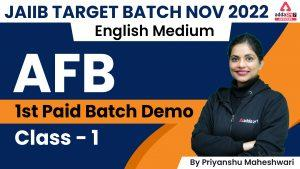Latest Banking jobs   »   Business Mathematics and Finance Notes

# Business Mathematics and Finance( Module A) Notes JAIIB AFB Exam 2022

Business Mathematics and Finance Notes: Business Mathematics and Finance Notes is for the candidates going to appear for the JAIIB Exam 2022. A number of numerical-based questions arise from this section in the JAIIB AFB Exam 2022. In this article, we have provided all the basic formulas which will be helpful to candidates in solving the numerical part. Business Mathematics and Finance Notes will be beneficial for the candidates at the time of revision as they can quickly go through the complete module and be updated.

## Business Mathematics and Finance Notes Unit 1: Calculation of Interest

Simple Interest: Interest calculated on the principal part of the loan is called Simple Interest. Also called as the flat rate. Amount of interest paid on the amount borrowed at a specified rate of interest and time period is known as simple interest. The formula for calculating simple interest is:

Interest= Principal*Rate*Time( PRT), in which

Interest – The total amount of interest paid
Principal- Amount that is lent or borrowed
Rate- The percentage of the principal charged as interest every year.
Time- Time for which the loan is borrowed.

Compound Interest: Compound Interest is the interest that is paid on the amount that includes the Principal and also the accumulated interests of the previous period. The Formula for calculating compound interest :

P = A(1 +r/n)nt,   in which

P – the principal
A – the amount deposited
r – the rate of interest
n = number of times per year that interest is compounded
t = number of years invested

Frequently compounding of Interest. If the interest is compounded :
Annually = P (1 + r)
Quarterly = P (1 + r/4)4
Monthly = P (1 + r/12)1

The Rule of 72: The Rule of 72 helps an investor in determining the number of years before their money doubles either in debt or investment. This can be done by dividing the number 72 by the percentage rate.

Equated Monthly Installments(EMIs): The monthly payment a borrower pays on his debt is called Equated Monthly Installments(EMI). The total of interest payment and principal repayment is called EMI. The total amount that is paid monthly is calculated in such a way that it remains constant all through the repayment tenure. For the fixed time of the loan the principal and the interest is paid through equal monthly installment. The benefit of EMI for borrowers is that they know exactly how much money they will have to pay each month for their loan and this will be easy for their personal budget.

Formula:

E = P*r*(1 + r)n/((1 + r)n – 1)

• E- EMI
• P- Principle Loan Amount
• r -is rate of interest
• n is tenure in number of months

Present Value: The price of a future sum of money in today’s time is called Present Value. Time, Expected rate of return, and the Size of the future cash flow are the main components of Present Value. One of the most fundamental and general concept in the finance subject is of Present Value. The basis of stock pricing, bond pricing, financial modeling, banking, insurance, pension fund valuation is the Present Value. Present value is mainly the time value of money and we can say that the money which we receive today can be invested today itself to receive a return.

The formula for calculating present value is:

PV = CF/(1+r)n

Here,
CF – cash flow in future period
r – the periodic rate of return or interest (also called the discount rate or the required rate of return)
n – number of periods

Future Value:  The method of calculating how much the present value (PV) of an asset or cash will be worth at a specific time in the future is called Future Value. There are two ways To calculate Future value there are 2 methods:

•  For an asset with simple annual interest: = Original Investment * (1+(interest rate*number of years))
• For an asset whose interest compounded annually: -Original Investment * ((1+interest rate)^number of years)

Annuities: A series of fixed payments required from you or paid to you at a specified frequency over the course of a fixed time period is called annuities. Payment Frequencies that are mostly used are yearly, semi-annually (twice a year), quarterly and monthly. The two basic types of annuities are: ordinary annuities and annuities due.

Ordinary Annuity: The annuities in which payment is required at the end of each period is called Ordinary Annuity. For example, straight bonds mostly pay coupon payments at the end of every six months until the date of bond maturity.

Annuity Due: The annuities in which payment is required at the starting of each period is called Annuity Due. One of the example of annuity due is rent. Rents are usually paid when someone moves first in the house at the beginning of the month, and then on the first of each month thereafter.

Present Value of an Annuity: The sum of the periodic payments each discounted at the given rate of interest to show the time value of money is called Present Value of an Annuity.

Present Value of an Ordinary Annuity = R (1 − (1 + i)-n)/i

Present Value of an Annuity Due = R (1 − (1 + i)-n)/i × (1 + i)

Here:

i – interest rate per compounding period
n-  number of compounding periods
R – fixed periodic payment.

## Business Mathematics and Finance Notes Unit 2: Calculation of Yield to Maturity( YTM)

Debt: An amount of money that a person owe to any other person is called Debt.  The most common examples of debt are loans from banks or financial institutions.

Bonds: Debt Capital means the amount that is borrowed and will be repaid at a particular date. Bonds and Debentures are the constituents of Debt Capital. Debt financial instruments issued by large corporations, financial institutions and government agencies that are backed up by collaterals or physical assets are called bonds. Debt financial instruments issued by private companies, but any collaterals or physical assets do not back them up are the debentures.

Some Important Terms Related to Bonds:

Face Value: Face value is the amount borrowed by a company and then repay the amount after a specific period. In other words Face Value is also known as par value. Face value is expressed on the face of the bond.

Coupon rate: Bonds have a specific rate of interest which is called the Coupon Rate.

Maturity: Maturity is the specific time period for which a bond is issued.

Redemption Value: Redemption value is the value that a bondholder gets on the maturity of the bond.  Bond is issued at a discount value and recovered at the par value.

Market Value: Bonds are traded on the stock exchange. Market value is the price at which the bond is generally bought or sold in the market.

Current Yield on Bond:

When the bond is purchased at its current market price and if the coupon interest is received then the measurement of the rate of return received from the bond is called Current Yield on Bond.

Current yield = Coupon interest/current market price

Yield to Maturity of Bond: Investors who purchases a bond and keeps it until its maturity period, the rate of return received is called Yield to Maturity of Bond.

## Business Mathematics and Finance Notes Unit 3: Capital Budgeting

The process used by a business to assess capable major projects or investments is called Capital Budgeting. There are certain techniques of capital budgeting such as: One has to choose between various projects; the life of a project includes Capital outflow(investment) and capital inflow(net profit); Present Value(PV) depends on the discount rate( cost of the capital); Present Value of all the cash inflows will be positive and of all the cash outflows will be negative; On total Present value of all the cash inflows and cash outflows is called Net Present Value(NPV); the discount rate at which net present value of a project is zero is called the Internal Rate of Return(IRR); Pay back period method is one of the other methods used for capital budgeting.

## Business Mathematics and Finance Notes Unit 4: Depreciation and its Accounting

Depreciation: Due to much usage, wear and tear the monetary value of an asset reduces. This reduction is termed as Depreciation. Long term assets are depreciated by businesses for both tax and accounting purposes. A number of factors lead to the depreciation of an asset such as unfavorable market conditions. Over a specified period of time the assets that can depreciate are equipment, currency and machinery. Different methods of Depreciation are: Straight line method (cost-residual value)/ useful life calculated; Declining balance method or writing down the value method where %age is fixed; Accelerated Depreciation and total of years’ digits method.

Depreciation is needed to know the profit correctly, get the correct financial position and make the equipment necessary for replacement of assets. Value and life of the asset and residual value are the elements of depreciation.

Related Posts:## FAQs: Business Mathematics and Finance Notes

Q.1 Where can we get the short notes of Business Mathematics and Finance for JAIIB AFB Exam 2022?

Ans. The short notes of Business Mathematics and Finance is mentioned in the article above.

Q.2 Which module of AFB mostly covers the numerical section?

Ans. Business Mathematics and Finance covers the most part of numerical section.#### Congratulations!Incorrect details? Fill the form again here

•Aiming for JAIIB NOV 2022? | Watch JAIIB...
•JAIIB AFB Topic Wise Weightage & Pre...
•IBPS RRB Clerk Prelims & Mains Onlin...
•IDBI Executive Previous Year Question Pa...
•IBPS RRB PO Prelims & Mains Online T...
•How long it takes to prepare for JAIIB?